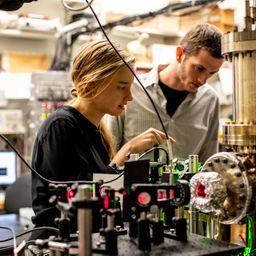## a. p=\sqrt{2 m q \Delta V}b. \frac{h}{\sqrt{2 m q \Delta V}}c. \text { see explanation }

Quantum Physics

Atomic Physics

### Discussion

You must be signed in to discuss.
##### Top Physics 103 Educators##### Christina K.

Rutgers, The State University of New Jersey

LB### Video Transcript

okay, We have a phenomenal to mystic particle and its accelerated through a certain potential difference. We want to get the momentum of the particle in terms of M Q and Delta Bi. So by energy conservation, you're gonna convert the potential energy gain by moving to the potential into kinetic energy. The potential energy of a particle that moves through potential Delta V is Q Delta V and that's equal to the change in kinetic energy. If it starts from rest, that's equal to 1/2. Um, b squared, Um, and another way you can write this is P squared over two. AM so P is equal to M B. Um and so this is equal to peace wherever two am If you kind of do the math out in your head, you can you know, you square this, get rid of that other factor of ram and divided by two. So then you got that p connecting this on DDE the two ends of the equation and you got P is equal to thio que Delta bi square rooted And yeah, so now we want to get into Berkeley wavelength. Um, so you can say that so this is our answer for a and then for beauty, you can say slams h overpay. So you just have to do h divided by this quantity is so its age divided by the square root of two. Um, que felt to be in what also they want, Um, if electronic protector of the same difference. Opposite directions Which particle have a shorter wavelength. Ah, interesting. So, um, let's go through this. So this is our quantity for the wavelength. You just gonna be the same? Two is obviously the same by the mass of the pro time. Um, yeah, the mass of the proton is gonna be bigger. All right? Yeah. Bigger. So, um, that's gonna make the wavelength smaller. So Lamda for the proton is gonna be less than the land up for the electron Just based on their masses. Um, yeah, yeah, that's hot. One worksUniversity of Washington

#### Topics

Quantum Physics

Atomic Physics

##### Top Physics 103 Educators##### Christina K.

Rutgers, The State University of New Jersey

LB